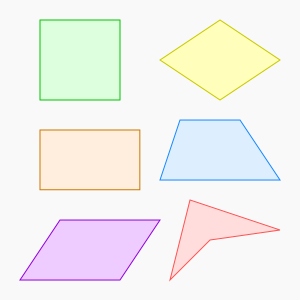Kids Encyclopedia FactsEdges and vertices 4
Schläfli symbol {4} (for square)
Area various methods;
see below
Internal angle (degrees) 90° (for square and rectangle)

In Euclidean plane geometry, a quadrilateral is a polygon with four edges (or sides) and four vertices or corners. Sometimes, the term quadrangle is used, by analogy with triangle, and sometimes tetragon for consistency with pentagon (5-sided), hexagon (6-sided) and so on.

The word "quadrilateral" is derived from the Latin words quadri, a variant of four, and latus, meaning "side".

Quadrilaterals are simple (not self-intersecting) or complex (self-intersecting), also called crossed. Simple quadrilaterals are either convex or concave.

All non-self-crossing quadrilaterals tile the plane by repeated rotation around the midpoints of their edges.Quadrilateral Facts for Kids. Kiddle Encyclopedia.